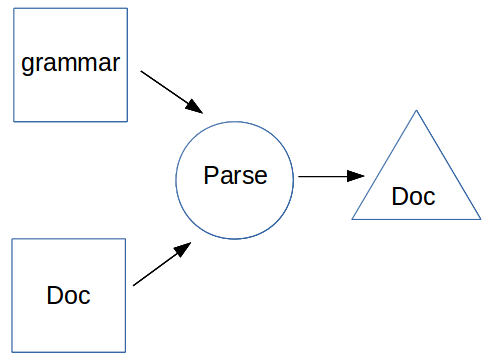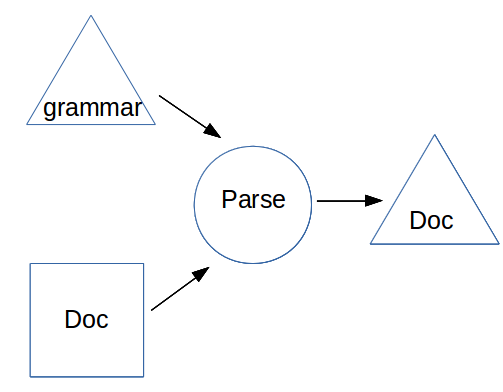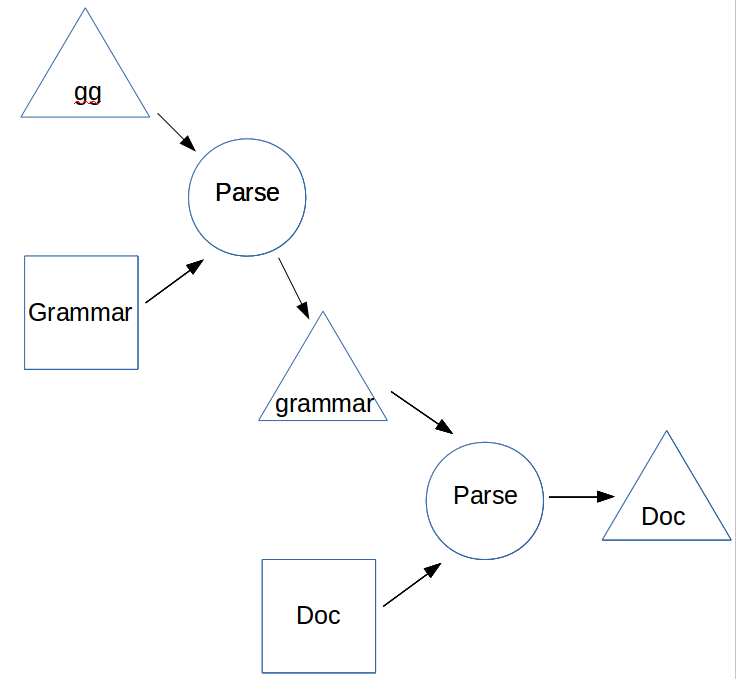# Data just wants to be (format) neutral

## Follow on to Balisage paper "Invisible XML"

"This is clearly a submission that needs to be shredded, burned, and the ashes buried in multiple locations"

"I think the audience will eat him alive. But I want to be there to hear it."

(Paper)

## All numbers are abstractions

Taking three as a number to reason about (a convenient number to state.)

3

The concept of "Three" is an abstraction: you can't point to "three", only three somethings.

## Representations

Given the right context, these all represent the same number:

• CXXVII
• 127
• 7F
• 1111111
• One hundred and twenty-seven
• Two to the power of seven, less one.

Which representations we choose depends on convenience, utility, familiarity, habit, context.

## Data

These are similarly equivalent:

`{"temperature": {"scale": "C"; "value": 21}}`

`<temperature scale="C" value="21"/>`

`<temperature scale="C">21</temperature>`

```<temperature>
<scale>C</scale>
<value>21</value>
</temperature>```

## XML

As I said: "Which representations we choose depends on convenience, utility, familiarity, habit, context."

One utility of XML is its generic data pipeline.

How do we resolve the conflicting requirements of convenience, utility, familiarity, habit, and context, and still enable a generic toolchain?

## Invisible XML

Allows you to inject any parsable structured document into the XML pipeline, and treat it as XML.

It is based on the observation that, looked at in the right way, an XML document is no more than the parse tree of some external form.

## Example

`a×(3+b)`

You could represent this in XML as

```<expr>
<prod>
<letter>a</letter>
<sum>
<digit>3</digit>
<letter>b</letter>
</sum>
</prod>
</expr>```

## Grammar

Let's take a suitable grammar for expressions:

```expr: term; sum; diff.
sum: expr, "+", term.
diff: expr, "-", term.
term: factor; prod; div.
prod: term, "×", factor.
div: term, "÷", factor.
factor: letter; digit; "(", expr, ")".
letter: ["a"-"z"].
digit: ["0"-"9"].```

## Parse tree of a×(3+b)

```      expr
|
term
|
prod
-----+------
|    |     |
term "×"  factor
|          |
factor   ----+-----
|      |   |    |
letter  "(" expr ")"
|           |
"a"         sum
-----+----
|    |   |
expr "+" term
|        |
term     factor
|        |
factor   letter
|        |
digit    "b"
|
"3"```

## Parse tree of a×(3+b)

```expr
|   term
|   |   prod
|   |   |   term
|   |   |   |   factor
|   |   |   |   |   letter
|   |   |   |   |   |   "a"
|   |   |  "×"
|   |   |   factor
|   |   |   |   "("
|   |   |   |   expr
|   |   |   |   |   sum
|   |   |   |   |   |   expr
|   |   |   |   |   |   |   term
|   |   |   |   |   |   |   |   factor
|   |   |   |   |   |   |   |   |   digit
|   |   |   |   |   |   |   |   |   |    "3"
|   |   |   |   |   |   "+"
|   |   |   |   |   |   term
|   |   |   |   |   |   |   factor
|   |   |   |   |   |   |   |   letter
|   |   |   |   |   |   |   |   |   "b"
|   |   |   |   ")"```

## Serialised as XML

```<expr>
<term>
<prod>
<term>
<factor>
<letter>a</letter>
</factor>
</term>
×
<factor>
(
<expr>
<sum>
<expr>
<term>
<factor>
<digit>3</digit>
</factor>
</term>
</expr>
+
<term>
<factor>
<letter>b</letter>
</factor>
</term>
</sum>
</expr>
)
</factor>
</prod>
</term>
</expr>```

## Marking the grammar

```expression: ^expr.
expr: term; ^sum; ^diff.
sum: expr, "+", term.
diff: expr, "-", term.
term: factor; ^prod; ^div.
prod: term, "×", factor.
div: term, "÷", factor.
factor: ^letter; ^digit; "(", expr, ")".
letter: ^["a"-"z"].
digit: ^["0"-"9"].```

## Serialising just the marked nodes

```<expr>
<prod>
<letter>a</letter>
<sum>
<digit>3</digit>
<letter>b</letter>
</sum>
</prod>
</expr>```

## Round-tripping

How to get back from the XML to the original format.

## Example: CSS

`body {color: blue; font-weight: bold}`

gives

```<css>
<rule>
<selector>body</selector>
<block>
<property>
<name>color</name>
<value>blue</value>
</property>
<property>
<name>font-weight</name>
<value>bold</value>
</property>
</block>
</rule>
</css>```

## Reserialising, with CSS

```block::before {content: "{"}
block::after {content: "}"}
name::after {content: ":"}
property::after {content: ";"}```

## Alternative

`body {color: blue; font-weight: bold}`

```<css>
<rule>
<selector>body</selector>
<block>
<property name="color" value="blue"/>
<property name="font-weight" value="bold"/>
</block>
</rule>
</css>```

```block::before {content: "{"}
block::after {content:"}"}
property::before {content: attr(name) ":" attr(value) ";"}```

## General case

Not possible, because of loss of context.

```<expr>
<prod>
<letter>a</letter>
<sum>
<digit>3</digit>
<letter>b</letter>
</sum>
</prod>
</expr>```

to

`a×(3+b)`

## Serialising the general parse tree

```serialise(t)=
for node in children(t):
select:
terminal(node):
output(node)
nonterminal(node):
serialise(node)```

## Reconstructing the original parse tree from the (reduced) parse tree

Walk through the reduced parse tree, hand in hand with the original grammar, reconstructing the original parse tree.

This is actually parsing, but rather than parsing text, we are parsing the (reduced) parse tree.

## Ambiguity

`<string>aaa</string>`

"aaa" vs 'aaa'

a+(3+b) vs a+((3+b))

## Condensing grammars

```expression: ^expr.
expr: term; ^sum; ^diff.
sum: expr, "+", term.
diff: expr, "-", term.
term: factor; ^prod; ^div.
prod: term, "×", factor.
div: term, "÷", factor.
factor: ^letter; ^digit; "(", expr, ")".
letter: ^["a"-"z"].
digit: ^["0"-"9"].```

to

```expr: operand.
sum: operand, operand.
diff: operand, operand.
prod: operand, operand.
div: operand, operand.
letter: ["a"-"z"].
digit: ["0"-"9"].```

where

`operand = (letter; digit; prod; div; sum; diff)`

## Diagram## Actually## ixml in ixml## Representational neutrality

```ixml: (^rule)+.
rule: @name, colon, definition, stop.
definition: (^alternative)+semicolon.
alternative: (term)*comma.
term: symbol; repetition.
...
name: (letter)+.
colon: ":".```

vs

```<ixml> ::= (^<rule>)+
<rule> ::= @<name> <define-symbol> <definition>
<definition> ::= (^<alternative>)+<bar>
<alternative> ::= (<term>)*
<term> ::= <symbol> | <repetition>
...
<name> ::= "<" (<letter>)+ ">"<define-symbol> ::= "::="
<bar> ::= "|"```

These have the same condensed grammars.

## Conversion

What this means is that as long as the reduced grammars are identical, you can convert between formats, by reading with one grammar, and writing with the other.

This also works for subsets, where one of the reduced grammars is a true subset of the other.

## Conclusion

In a sense ixml is an 'obvious' idea. But I suspect that it is obvious only once you have heard it.

I now know of four implementations. Please tell me if you implement it, and give me feedback!